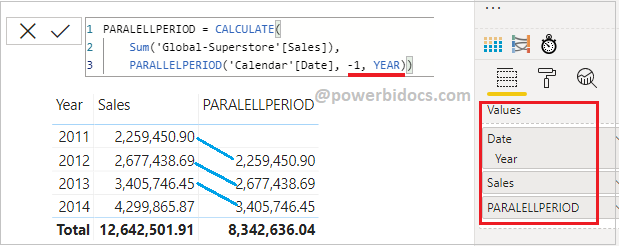Home » DAX » DAX PARALLELPERIOD

# DAX PARALLELPERIODDAX PARALLELPERIOD function returns a table that contains a column of dates that represents a period parallel to the dates in the specified dates column, in the current context, with the dates shifted a number of intervals either forward in time or back in time.

It comes under Time Intelligence DAX function category.

#### Syntax:

```PARALLELPERIOD(<dates>,<number_of_intervals>,<interval>)
```

#### Parameters:

dates: A column that contains dates.

number of intervals: An integer that specifies the number of intervals to add to or subtract from the dates.

interval: The interval by which to shift the dates. The value for interval can be one of the following: year, quarter, month.

Note: After load data into Power BI file, check the datatype for order date column, if datatype is not in date format then change it as in date.

Step-1: Create a calendar table to using existing dataset “Order Date” column.

Go to Modeling Tab > Click to table icon & write below DAX

```Calendar = CALENDAR(
MIN('Global-Superstore'[Order Date]),
MAX('Global-Superstore'[Order Date]))```

Step-2: After that create a relationship between both table.Relationship

Step-3: Now create a measure to get last year sale.

```PARALELLPERIOD = CALCULATE(
Sum('Global-Superstore'[Sales]),
PARALLELPERIOD('Calendar'[Date], -1, YEAR))```

Step-4:  Add table visual into report page and drag Year & month column from calendar table,  sales from Global-Superstore table and new created measure.DAX Paralellperiod function

Now you can see in below screen shot – Against each rows of 2012 it returns total sales of 2011 and for year 2013 it returns total sales of 2012.

Because in DAX formula we passed parameter values – number_of_interval: -1 & interval: Year. That’s why it is returning one year back sale.Paralellperiod Dax example in Power BI

#### Let’s try with year column-Paralellperiod dax eample-1

Now change the DAX parameter value for number_of_interval = 1, so you can see the result, it is showing next year sale against each year.Paralellperiod dax example -2

Hope you enjoyed the post. Your valuable feedback, question, or comments about this post are always welcome or you can leave us message on our contact form , we will revert to you asap.

## 3 thoughts on “DAX PARALLELPERIOD”

1.Try to cover rank, earlier, dateadd and others functions also your explanation is amazing..

1.Thanks Piyush.

2.Hi,

Great Content! Just one small request – could you please post a step-by-step working of this formula as well:

CALCULATE(
Sum(‘Global-Superstore'[Sales]),
PARALLELPERIOD(‘Calendar'[Date], -1, YEAR))

Thanks!